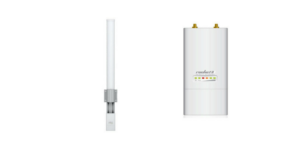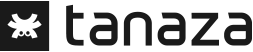+39 02 8718 8553 sales@tanaza.com
Select Page

# Understand the power gain with the Rule of 10s and 3s

Jun 30, 2017

In the datasheet of your access points, you will find the device’s radio power. This value describes how well the transmitting antenna converts the input power into radio waves (output power). This is also called “power gain”.The power of an access point is the intensity of its wireless signal. In general, people  believe that the higher the power, the further distance you can cover. But this is not true, as the mathematical relation between the covered area and the power of the access point is much more subtle.

Before explaining the formulas, let’s make a practical example.

Imagine that the intensity of the wireless signal is represented by a light bulb.
If you light the bulb in a dark room, the room will be equally illuminated by the bulb, in all directions with the same intensity.

In contrast, if you use a mirror to give a direction to the light, its intensity will be higher. Watch this video for more details.This change in intensity is what is called the gain; it represents the increase of power, in other words the ratio between the input power and the output power.

Gain = Power output / Power input

The gain of antennas is expressed in decibels (dB). In the wireless communication sector, the power output and input are in milliWatts (mW).

To be simple, let’s see the so-called Rule of 10s and 3s, that allows us to calculate output power of an access point.

• For every gain of 3 dB, the power in mW is doubled
• For every loss of 3 dB, the power in mW is halved
• For every gain of 10 dB, the power in mW is multiplied by 10
• For every loss of 10 dB, the power in mW is divided by 10

Example: we have an access point that can transmit 100 mW. We add an antenna that it supposes to give a gain of 3 dB.

100 mW + 3 dB = 100 x 2 = 200 mW

To conclude, if you want to optimise your WLAN performances, you have to think carefully about the power level you need and the direction in which you want to spread the wireless signal. Prefer mono-directional antennas if you want to cover a small specific area, rather than an access point with omnidirectional antennas for bigger deployment.

If you are interested in buying antenna for your access point, here one model of antenna that you can use with a Ubiquiti Rocket M2Here a video about how to installed an antenna  with an Outdoor UniFi.

##### COMPANYLegal Information Tanaza S.p.A., via Carlo de Cristoforis 13, 20124 Milano, Italy - VAT Number: IT07081410966This project has received funding from the European Union’s Horizon 2020 research and innovation programme under grant agreement No 873466.